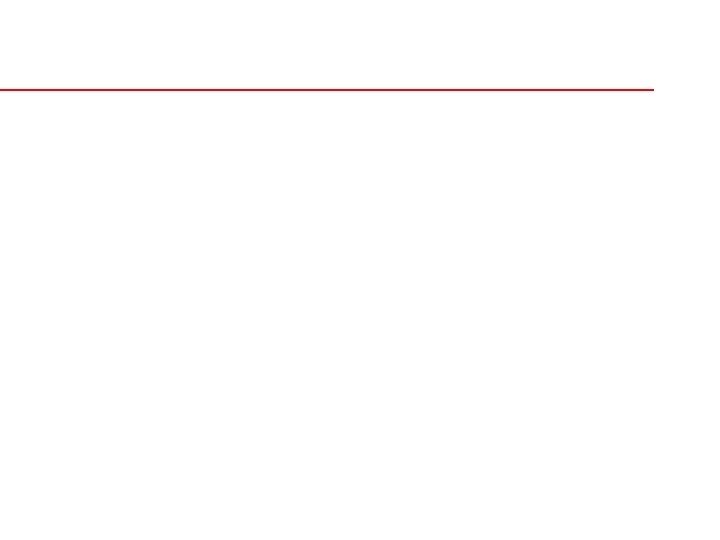Скачать презентацию IV Administration Dosage Calculation Keith Rischer RN

18a728f47945fa2b022927e835635e20.ppt

• Количество слайдов: 30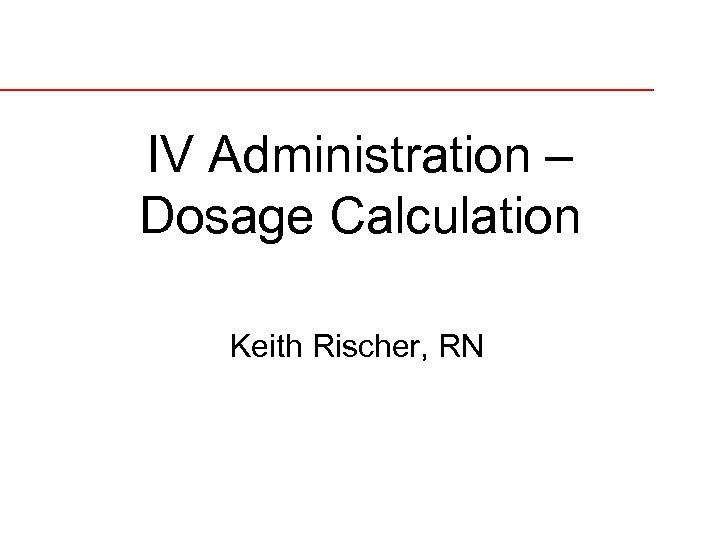IV Administration – Dosage Calculation Keith Rischer, RN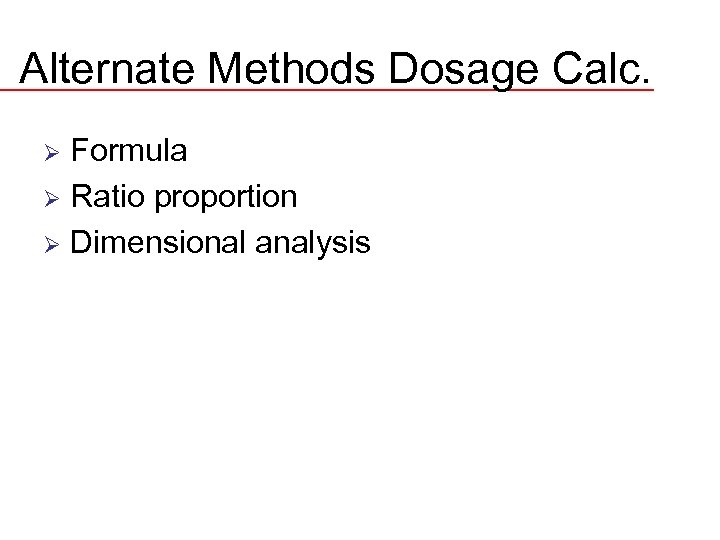Alternate Methods Dosage Calc. Formula Ø Ratio proportion Ø Dimensional analysis Ø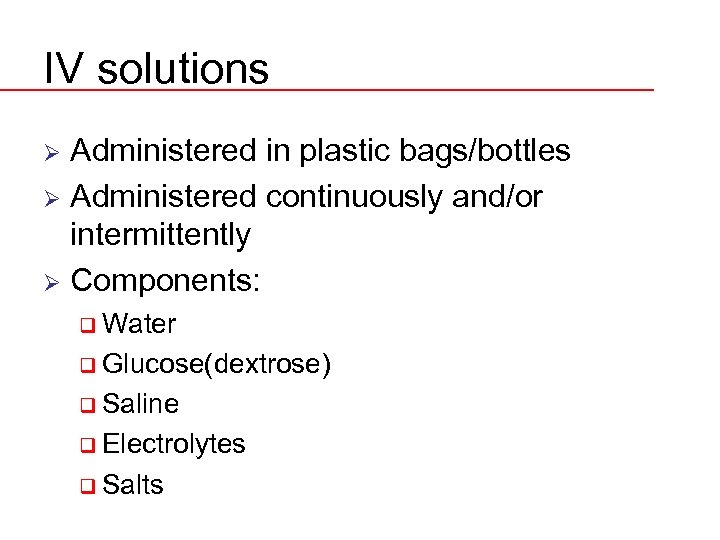IV solutions Administered in plastic bags/bottles Ø Administered continuously and/or intermittently Ø Components: Ø q Water q Glucose(dextrose) q Saline q Electrolytes q Salts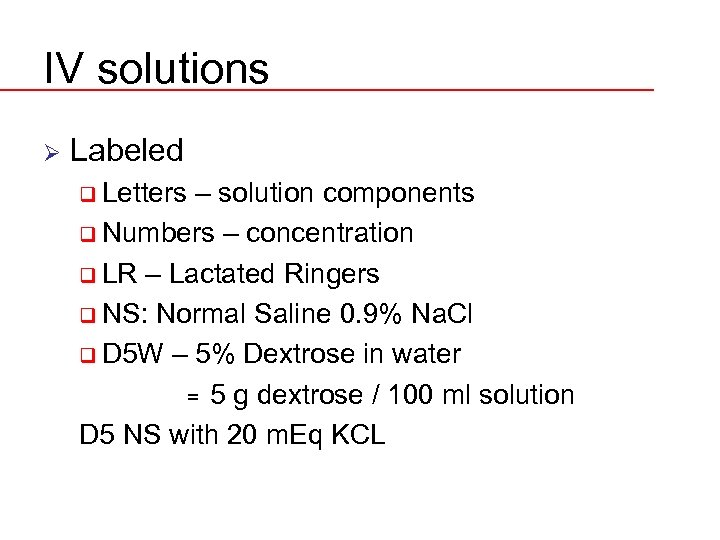IV solutions Ø Labeled q Letters – solution components q Numbers – concentration q LR – Lactated Ringers q NS: Normal Saline 0. 9% Na. Cl q D 5 W – 5% Dextrose in water = 5 g dextrose / 100 ml solution D 5 NS with 20 m. Eq KCL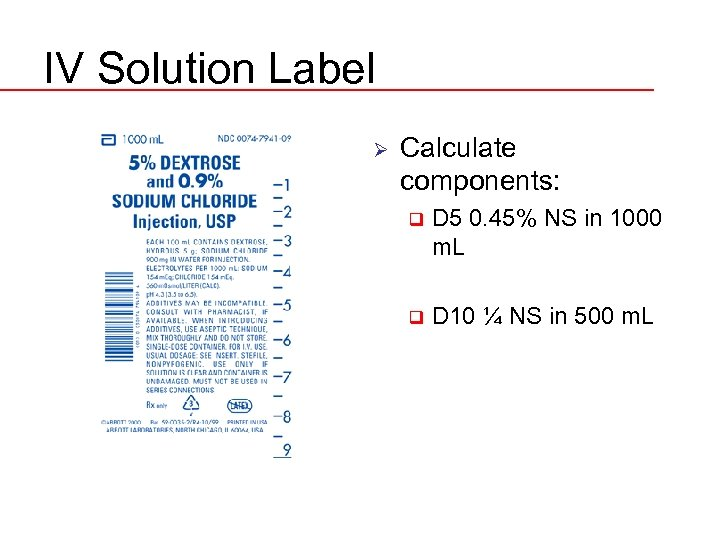IV Solution Label Ø Calculate components: q D 5 0. 45% NS in 1000 m. L q D 10 ¼ NS in 500 m. L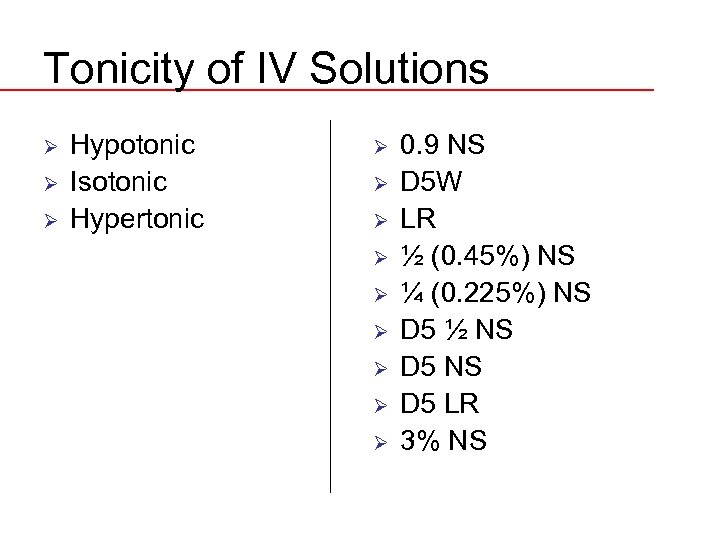Tonicity of IV Solutions Ø Ø Ø Hypotonic Isotonic Hypertonic Ø Ø Ø Ø Ø 0. 9 NS D 5 W LR ½ (0. 45%) NS ¼ (0. 225%) NS D 5 ½ NS D 5 LR 3% NS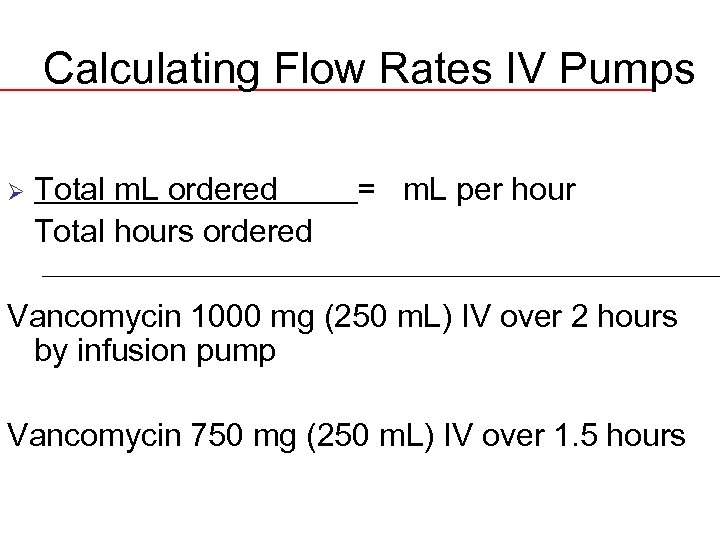Calculating Flow Rates IV Pumps Ø Total m. L ordered Total hours ordered = m. L per hour Vancomycin 1000 mg (250 m. L) IV over 2 hours by infusion pump Vancomycin 750 mg (250 m. L) IV over 1. 5 hours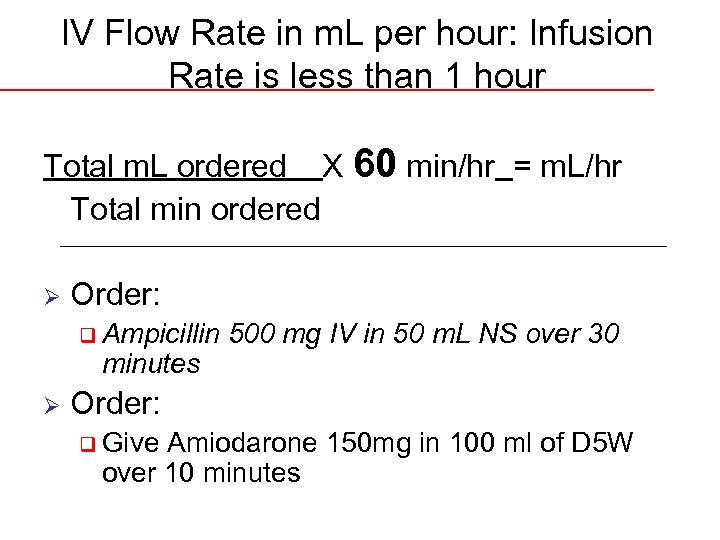IV Flow Rate in m. L per hour: Infusion Rate is less than 1 hour Total m. L ordered X 60 min/hr = m. L/hr Total min ordered Ø Order: q Ampicillin minutes Ø 500 mg IV in 50 m. L NS over 30 Order: q Give Amiodarone 150 mg in 100 ml of D 5 W over 10 minutes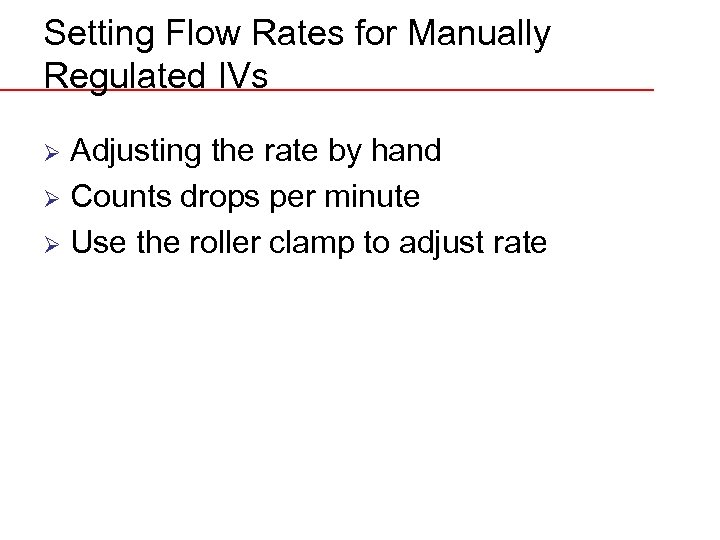Setting Flow Rates for Manually Regulated IVs Adjusting the rate by hand Ø Counts drops per minute Ø Use the roller clamp to adjust rate Ø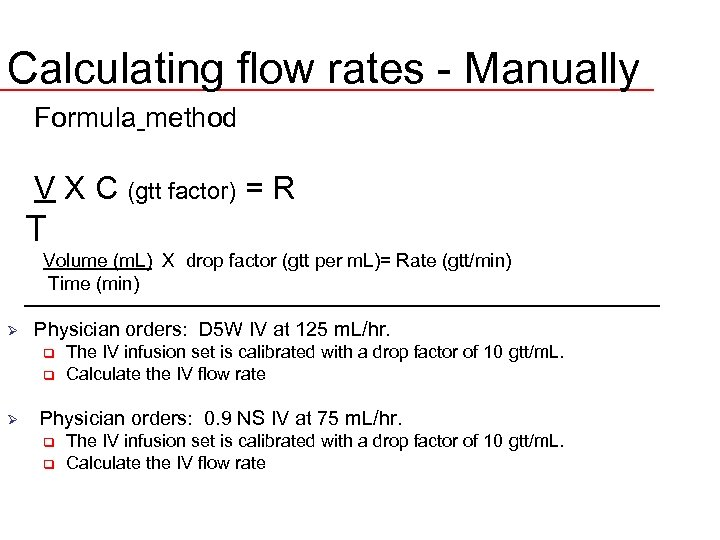Calculating flow rates - Manually Formula method V X C (gtt factor) = R T Volume (m. L) X drop factor (gtt per m. L)= Rate (gtt/min) Time (min) Ø Physician orders: D 5 W IV at 125 m. L/hr. q q Ø The IV infusion set is calibrated with a drop factor of 10 gtt/m. L. Calculate the IV flow rate Physician orders: 0. 9 NS IV at 75 m. L/hr. q q The IV infusion set is calibrated with a drop factor of 10 gtt/m. L. Calculate the IV flow rate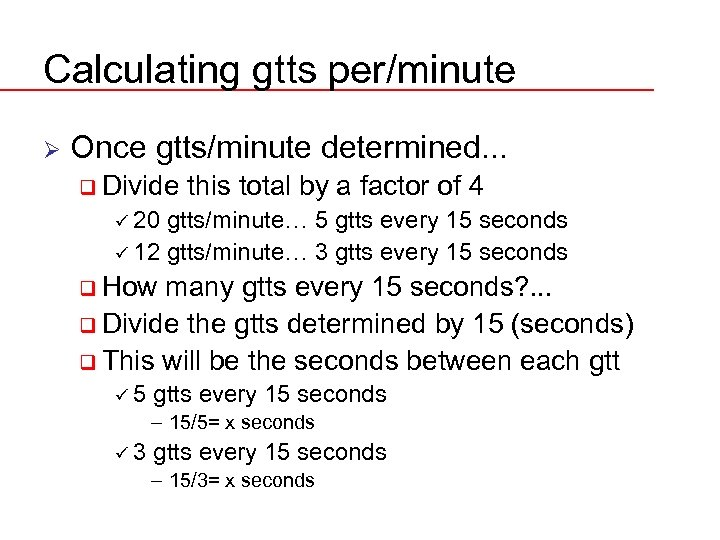Calculating gtts per/minute Ø Once gtts/minute determined. . . q Divide this total by a factor of 4 ü 20 gtts/minute… 5 gtts every 15 seconds ü 12 gtts/minute… 3 gtts every 15 seconds q How many gtts every 15 seconds? . . . q Divide the gtts determined by 15 (seconds) q This will be the seconds between each gtt ü 5 gtts every 15 seconds – 15/5= x seconds ü 3 gtts every 15 seconds – 15/3= x seconds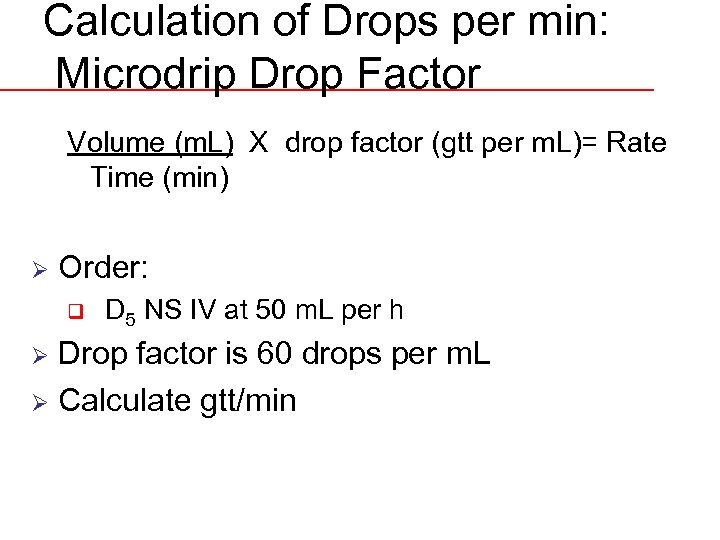Calculation of Drops per min: Microdrip Drop Factor Volume (m. L) X drop factor (gtt per m. L)= Rate Time (min) Ø Order: q D 5 NS IV at 50 m. L per h Drop factor is 60 drops per m. L Ø Calculate gtt/min Ø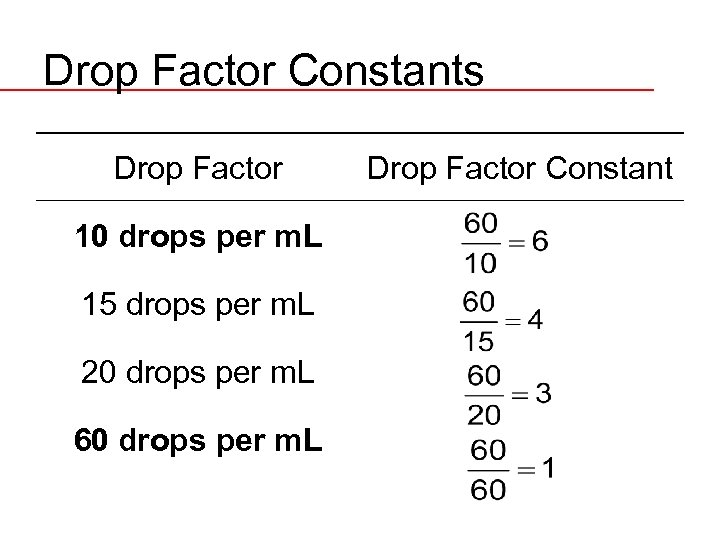Drop Factor Constants Drop Factor 10 drops per m. L 15 drops per m. L 20 drops per m. L 60 drops per m. L Drop Factor Constant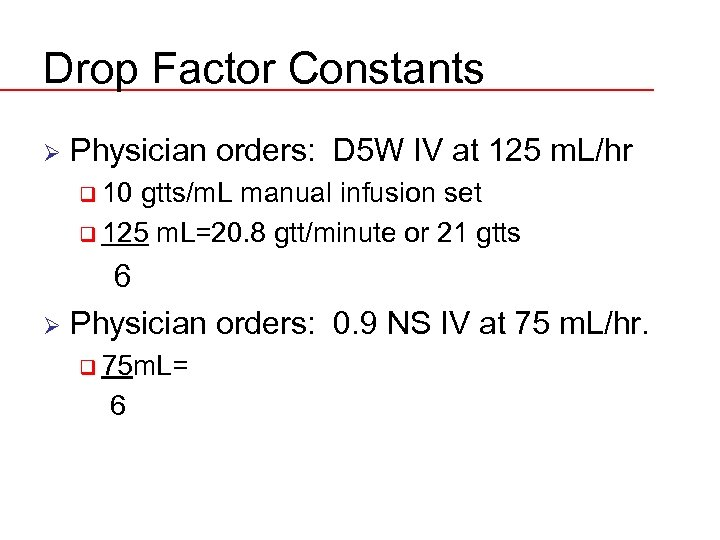Drop Factor Constants Ø Physician orders: D 5 W IV at 125 m. L/hr q 10 gtts/m. L manual infusion set q 125 m. L=20. 8 gtt/minute or 21 gtts 6 Ø Physician orders: 0. 9 NS IV at 75 m. L/hr. q 75 m. L= 6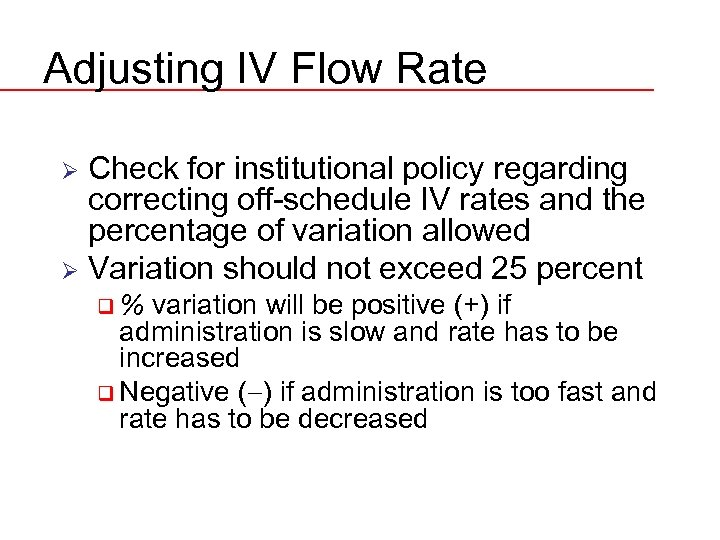Adjusting IV Flow Rate Check for institutional policy regarding correcting off-schedule IV rates and the percentage of variation allowed Ø Variation should not exceed 25 percent Ø q% variation will be positive (+) if administration is slow and rate has to be increased q Negative ( ) if administration is too fast and rate has to be decreased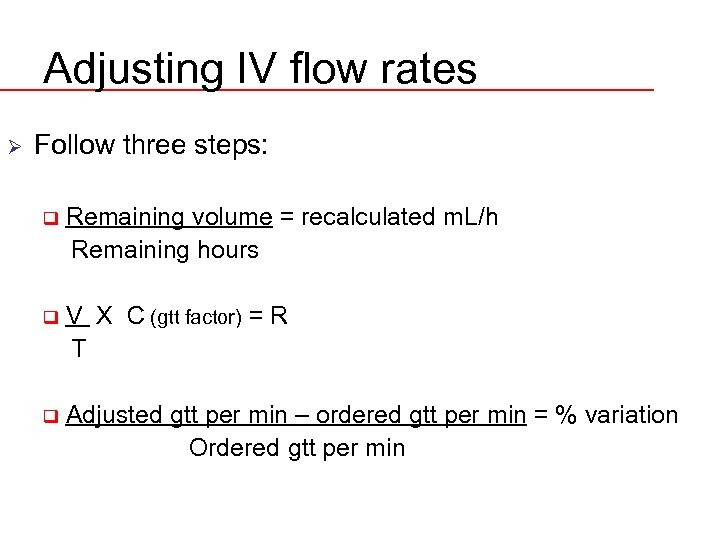Adjusting IV flow rates Ø Follow three steps: q Remaining volume = recalculated m. L/h Remaining hours q V X C (gtt factor) = R T q Adjusted gtt per min – ordered gtt per min = % variation Ordered gtt per min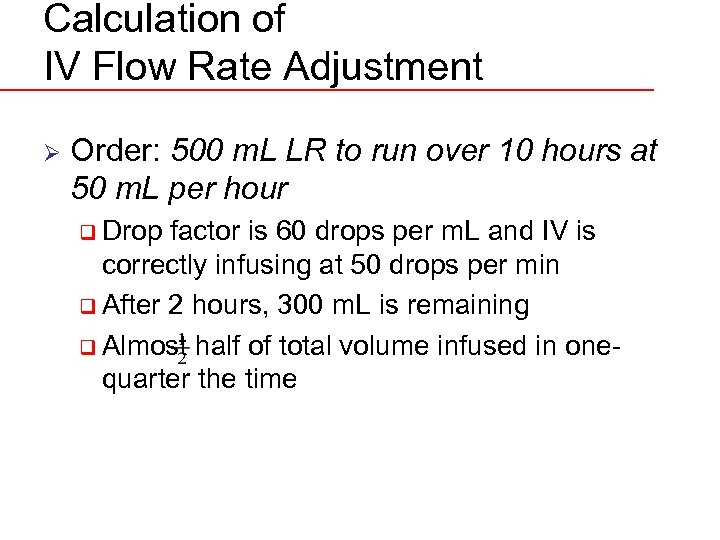Calculation of IV Flow Rate Adjustment Ø Order: 500 m. L LR to run over 10 hours at 50 m. L per hour q Drop factor is 60 drops per m. L and IV is correctly infusing at 50 drops per min q After 2 hours, 300 m. L is remaining q Almost half of total volume infused in onequarter the time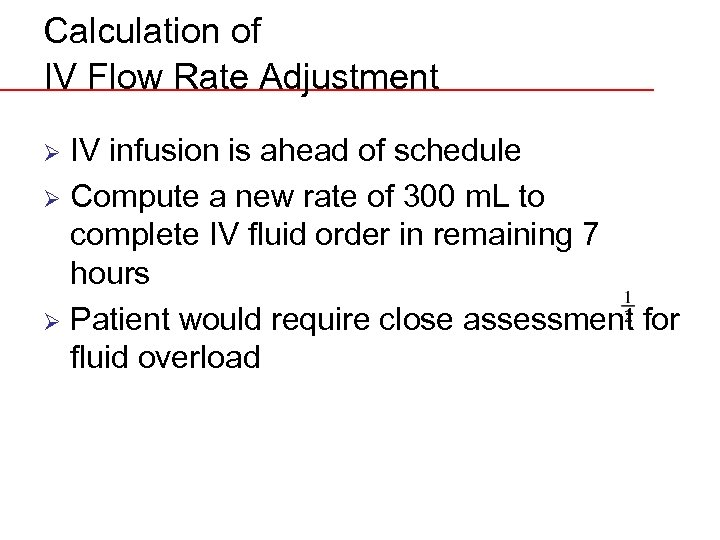Calculation of IV Flow Rate Adjustment IV infusion is ahead of schedule Ø Compute a new rate of 300 m. L to complete IV fluid order in remaining 7 hours Ø Patient would require close assessment for fluid overload Ø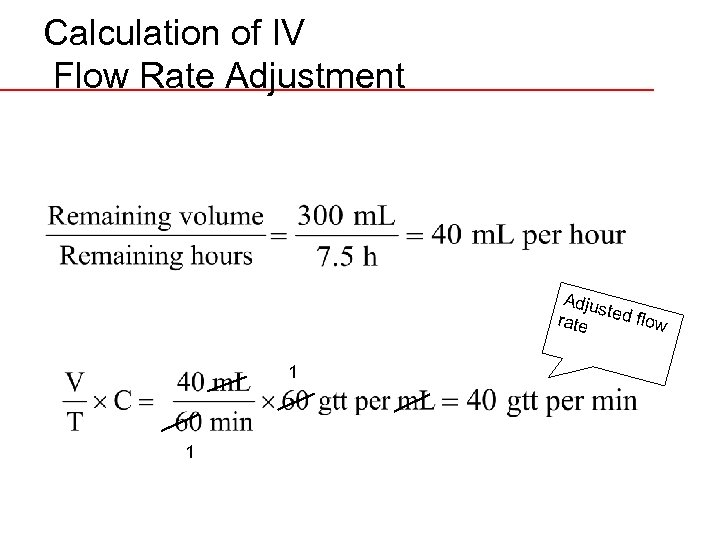Calculation of IV Flow Rate Adjustment Adju st rate ed flow 1 1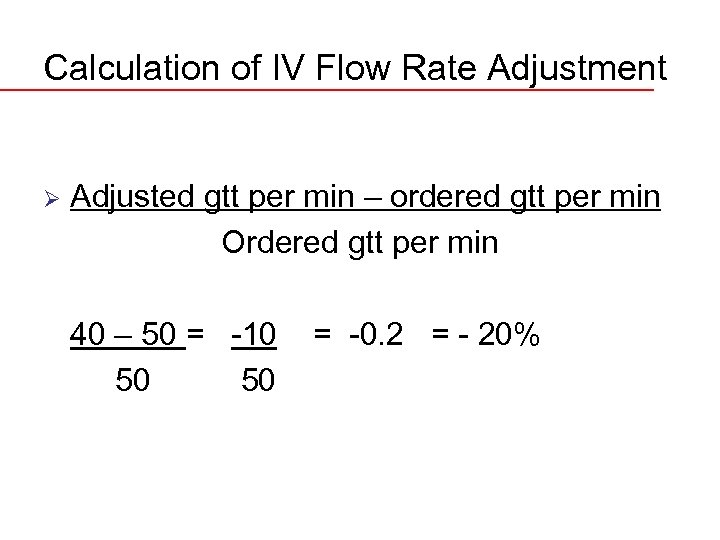Calculation of IV Flow Rate Adjustment Ø Adjusted gtt per min – ordered gtt per min Ordered gtt per min 40 – 50 = -10 50 50 = -0. 2 = - 20%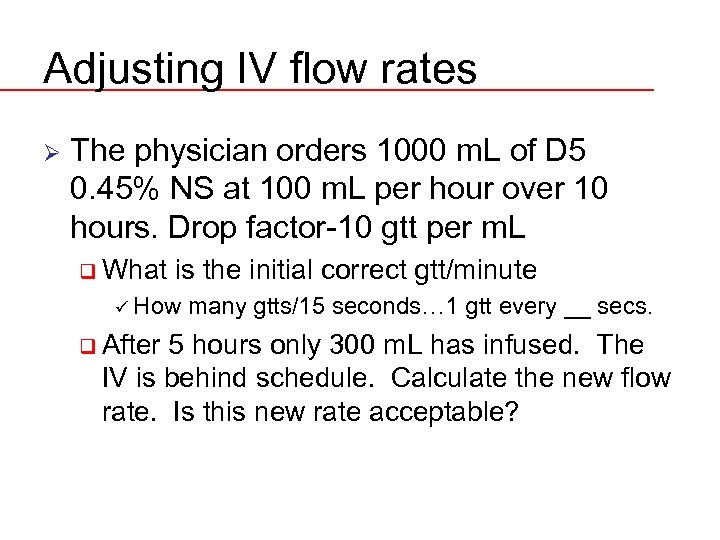Adjusting IV flow rates Ø The physician orders 1000 m. L of D 5 0. 45% NS at 100 m. L per hour over 10 hours. Drop factor-10 gtt per m. L q What is the initial correct gtt/minute ü How q After many gtts/15 seconds… 1 gtt every __ secs. 5 hours only 300 m. L has infused. The IV is behind schedule. Calculate the new flow rate. Is this new rate acceptable?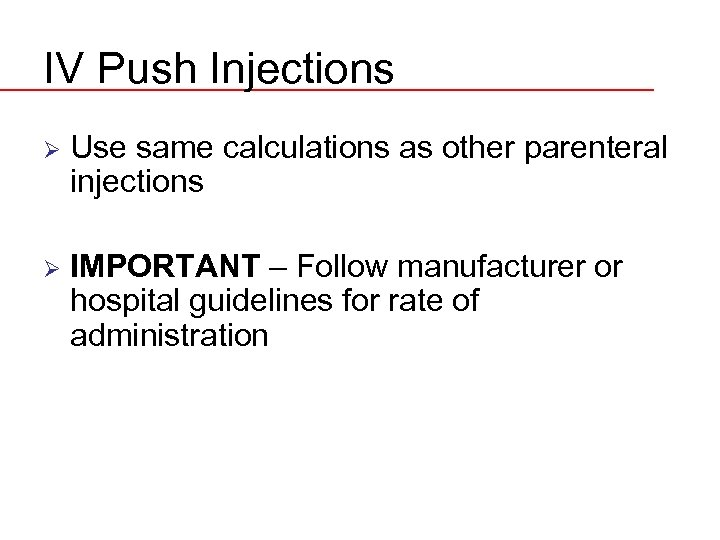IV Push Injections Ø Use same calculations as other parenteral injections Ø IMPORTANT – Follow manufacturer or hospital guidelines for rate of administration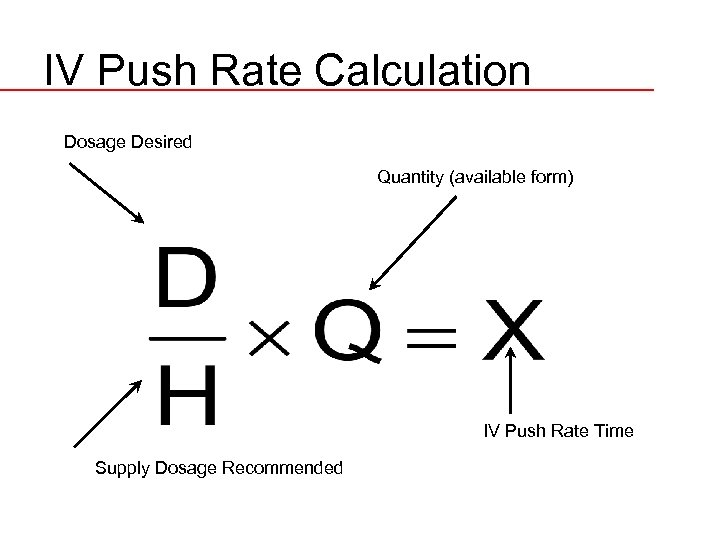IV Push Rate Calculation Dosage Desired Quantity (available form) IV Push Rate Time Supply Dosage Recommended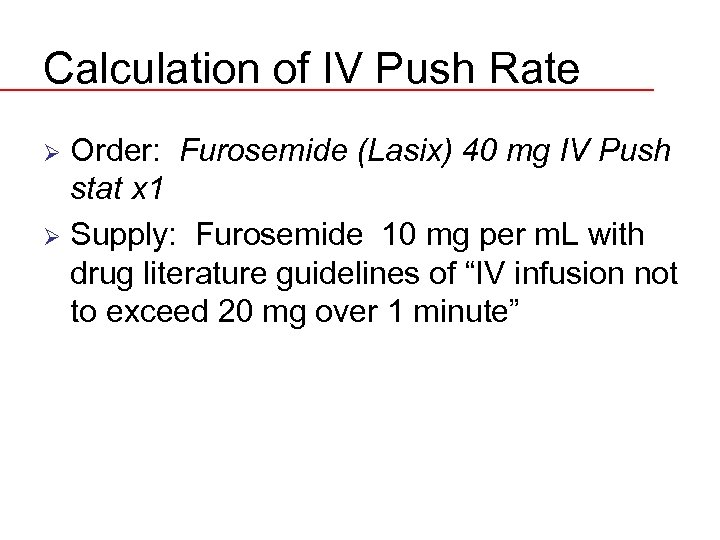Calculation of IV Push Rate Order: Furosemide (Lasix) 40 mg IV Push stat x 1 Ø Supply: Furosemide 10 mg per m. L with drug literature guidelines of “IV infusion not to exceed 20 mg over 1 minute” Ø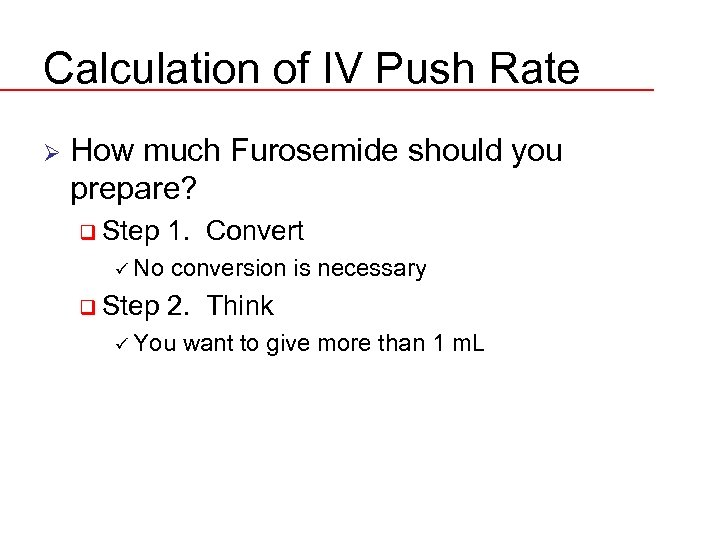Calculation of IV Push Rate Ø How much Furosemide should you prepare? q Step ü No q Step 1. Convert conversion is necessary 2. Think ü You want to give more than 1 m. L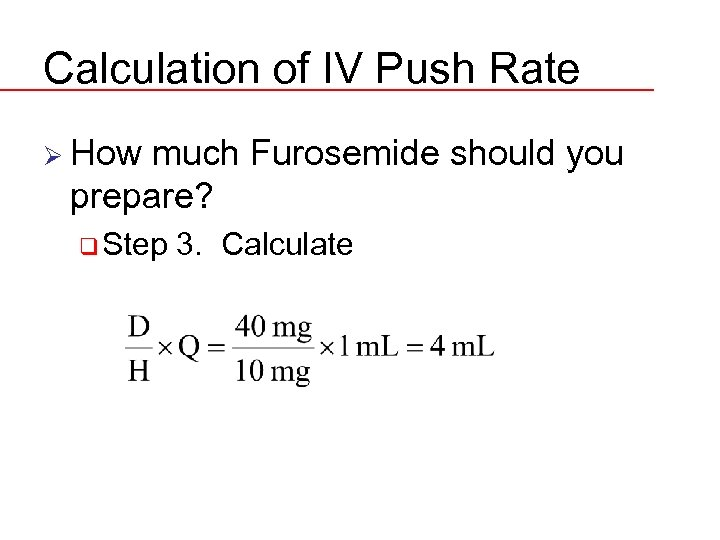Calculation of IV Push Rate Ø How much Furosemide should you prepare? q Step 3. Calculate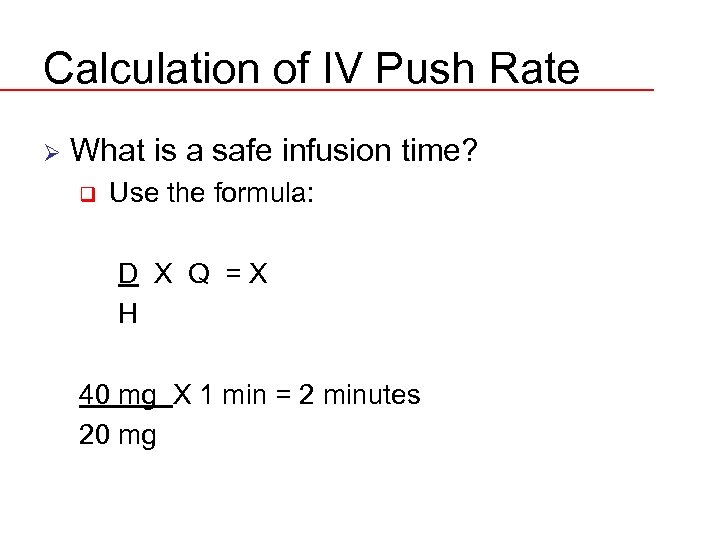Calculation of IV Push Rate Ø What is a safe infusion time? q Use the formula: D X Q =X H 40 mg X 1 min = 2 minutes 20 mg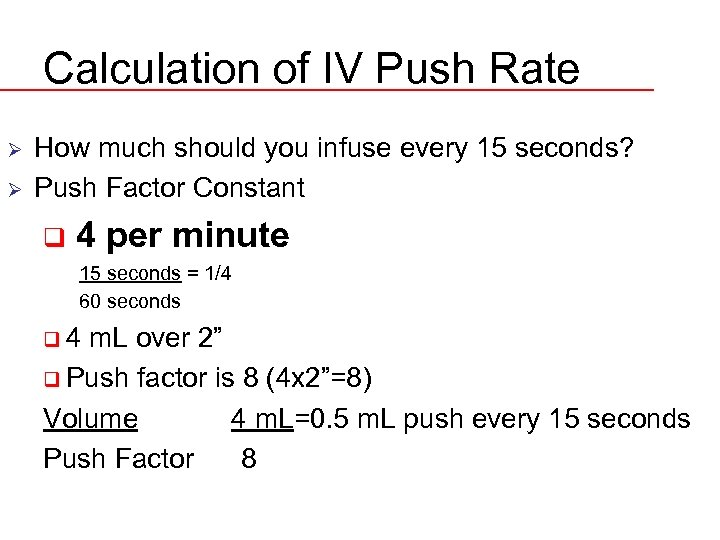Calculation of IV Push Rate Ø Ø How much should you infuse every 15 seconds? Push Factor Constant q 4 per minute 15 seconds = 1/4 60 seconds q 4 m. L over 2” q Push factor is 8 (4 x 2”=8) Volume 4 m. L=0. 5 m. L push every 15 seconds Push Factor 8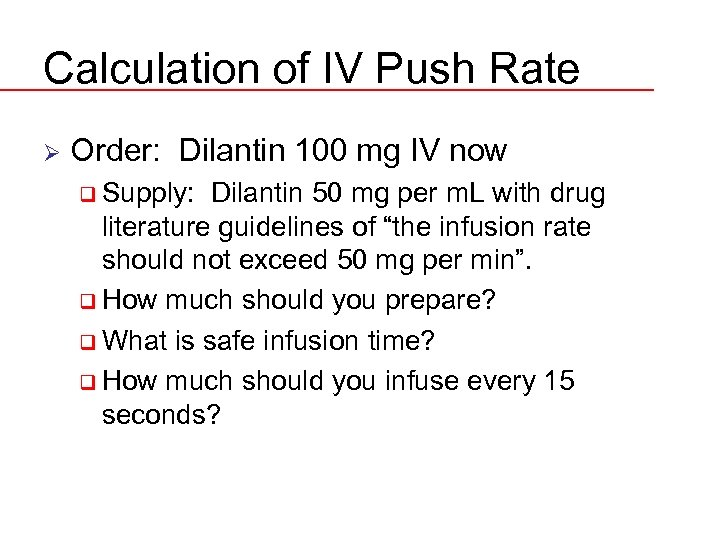Calculation of IV Push Rate Ø Order: Dilantin 100 mg IV now q Supply: Dilantin 50 mg per m. L with drug literature guidelines of “the infusion rate should not exceed 50 mg per min”. q How much should you prepare? q What is safe infusion time? q How much should you infuse every 15 seconds?